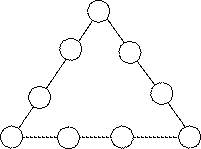# Magic Triangle

Given the “magic triangle” shown, place the digits 1 through 9 in the circles in a very special way, using each digit once and only once. For each side of the triangle, the sum of the two “inside circles” subtracted from the sum of the two “end circles” must be the same “magic number.” Find the magic triangles whose magic numbers are 0, 3, and 9.(Source: Mathematics Teaching in the Middle School, Sep-Oct 1994)